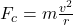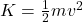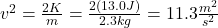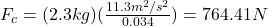## Say you want to make a sling by swinging a mass M of 2.3 kg in a horizontal circle of radius 0.034 m, using a string of length 0.034 m. You

Question

Say you want to make a sling by swinging a mass M of 2.3 kg in a horizontal circle of radius 0.034 m, using a string of length 0.034 m. You wish the mass to have a kinetic energy of 13.0 Joules when released. How strong will the string need to be

in progress 0
2 weeks 2021-09-02T20:05:23+00:00 1 Answers 0 views 0

T = 764.41 N

Explanation:

In this case the tension of the string is determined by the centripetal force. The formula to calculate the centripetal force is given by:(1)

m: mass object = 2.3 kg

r: radius of the circular orbit = 0.034 m

v: tangential speed of the object

However, it is necessary to calculate the velocity v first. To find v you use the formula for the kinetic energy:You have the value of the kinetic energy (13.0 J), then, you replace the values of K and m, and solve for v^2:you replace this value of v in the equation (1). Also, you replace the values of r and m:hence, the tension in the string must be T =  Fc = 764.41 N